Скачать презентацию Minimax Estimators Dominating the Least-Squares Estimator Zvika Ben-Haim

817406fd00cac03678e277796476bfc6.ppt

• Количество слайдов: 16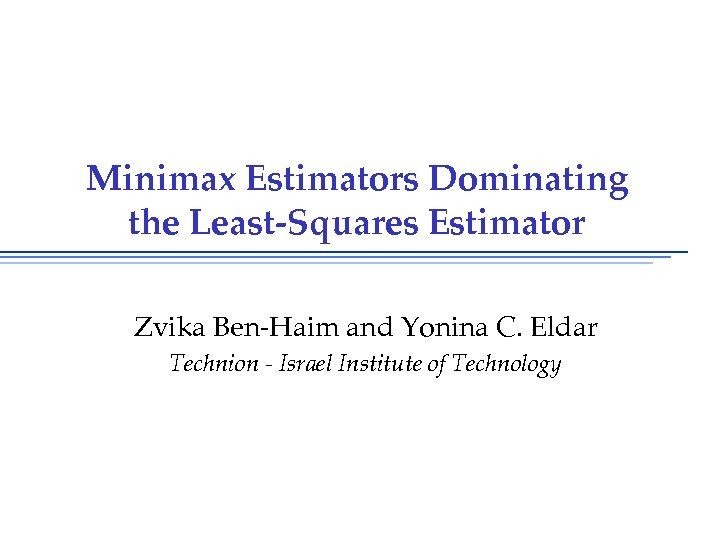Minimax Estimators Dominating the Least-Squares Estimator Zvika Ben-Haim and Yonina C. Eldar Technion - Israel Institute of Technology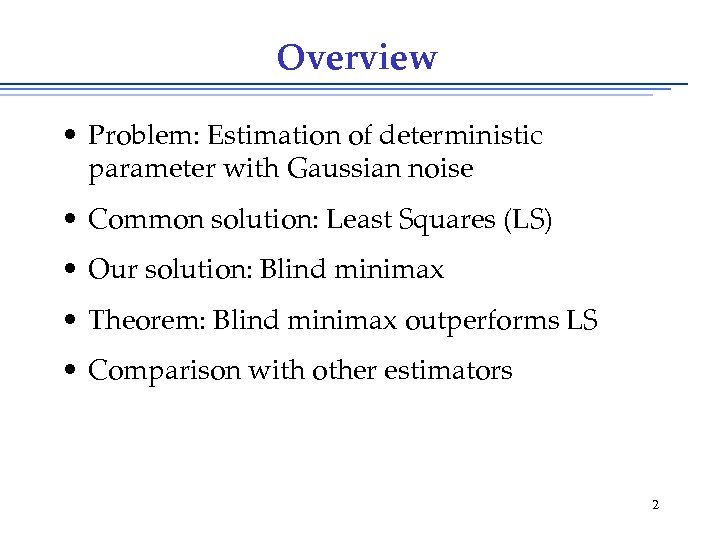Overview • Problem: Estimation of deterministic parameter with Gaussian noise • Common solution: Least Squares (LS) • Our solution: Blind minimax • Theorem: Blind minimax outperforms LS • Comparison with other estimators 2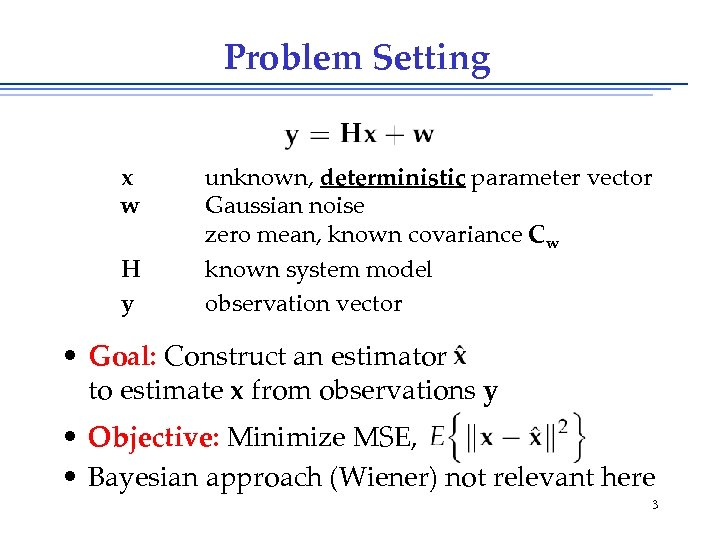Problem Setting x w H y unknown, deterministic parameter vector Gaussian noise zero mean, known covariance Cw known system model observation vector • Goal: Construct an estimator x to estimate x from observations y • Objective: Minimize MSE, • Bayesian approach (Wiener) not relevant here 3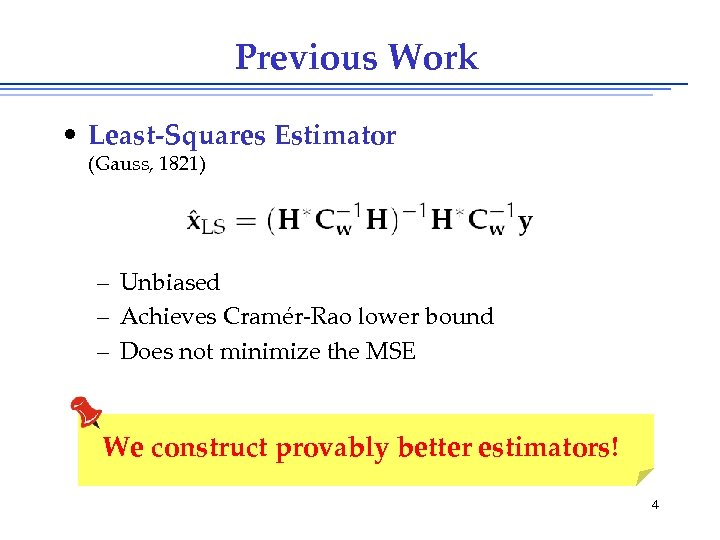Previous Work • Least-Squares Estimator (Gauss, 1821) – Unbiased – Achieves Cramér-Rao lower bound – Does not minimize the MSE We construct provably better estimators! 4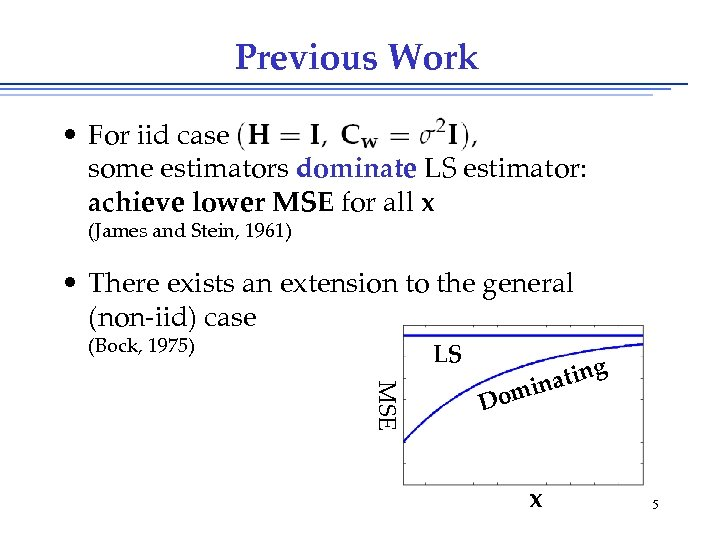Previous Work • For iid case some estimators dominate LS estimator: achieve lower MSE for all x (James and Stein, 1961) • There exists an extension to the general (non-iid) case (Bock, 1975) LS MSE g in inat om D x 5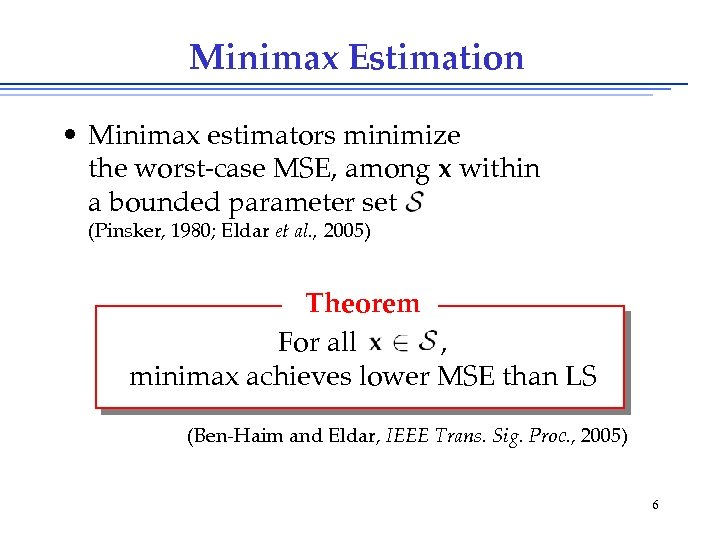Minimax Estimation • Minimax estimators minimize the worst-case MSE, among x within a bounded parameter set (Pinsker, 1980; Eldar et al. , 2005) Theorem For all , minimax achieves lower MSE than LS (Ben-Haim and Eldar, IEEE Trans. Sig. Proc. , 2005) 6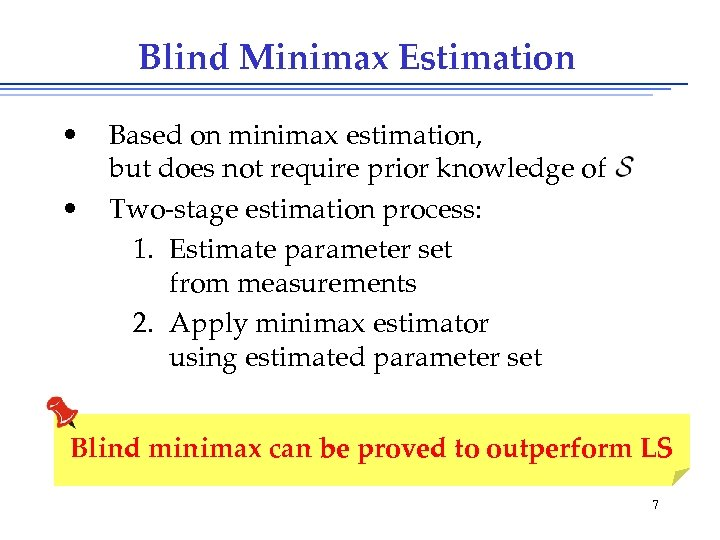Blind Minimax Estimation • • Based on minimax estimation, but does not require prior knowledge of Two-stage estimation process: 1. Estimate parameter set from measurements 2. Apply minimax estimator using estimated parameter set Blind minimax can be proved to outperform LS 7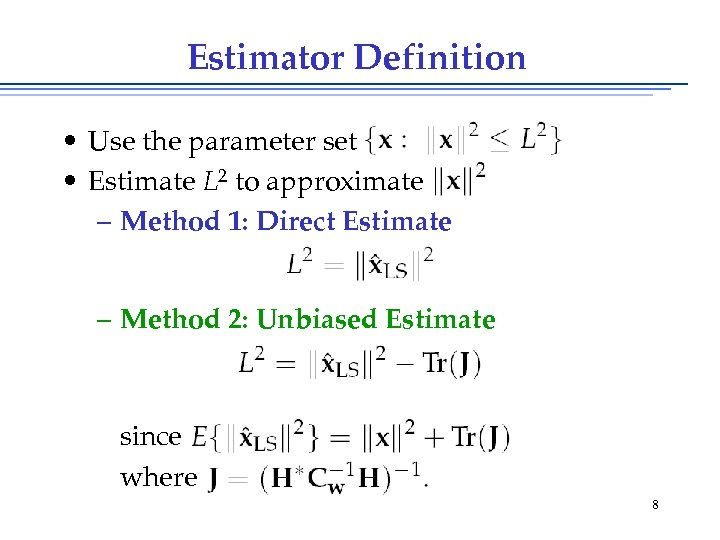Estimator Definition • Use the parameter set • Estimate L 2 to approximate – Method 1: Direct Estimate – Method 2: Unbiased Estimate since where 8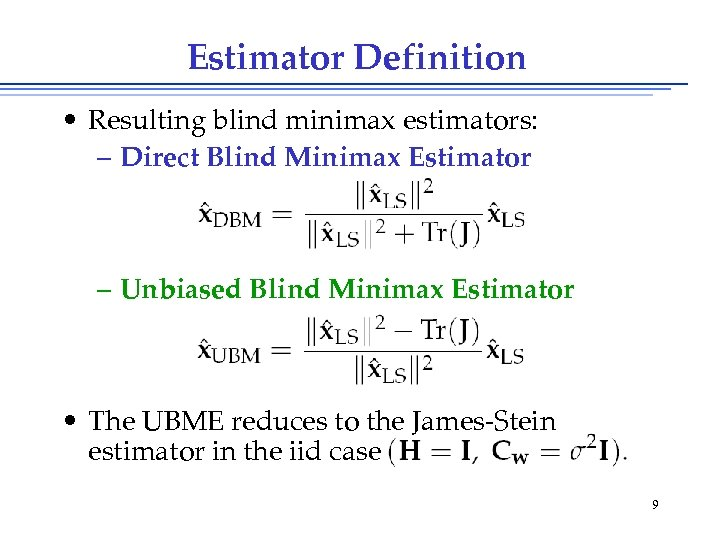Estimator Definition • Resulting blind minimax estimators: – Direct Blind Minimax Estimator – Unbiased Blind Minimax Estimator • The UBME reduces to the James-Stein estimator in the iid case 9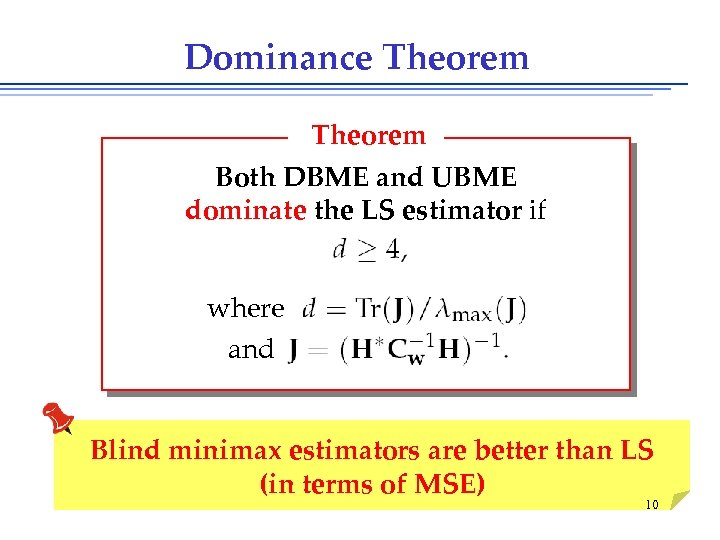Dominance Theorem Both DBME and UBME dominate the LS estimator if where and Blind minimax estimators are better than LS (in terms of MSE) 10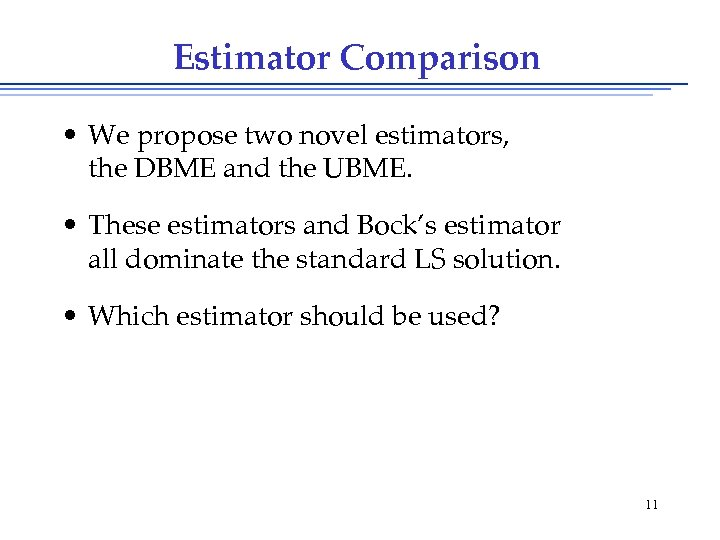Estimator Comparison • We propose two novel estimators, the DBME and the UBME. • These estimators and Bock’s estimator all dominate the standard LS solution. • Which estimator should be used? 11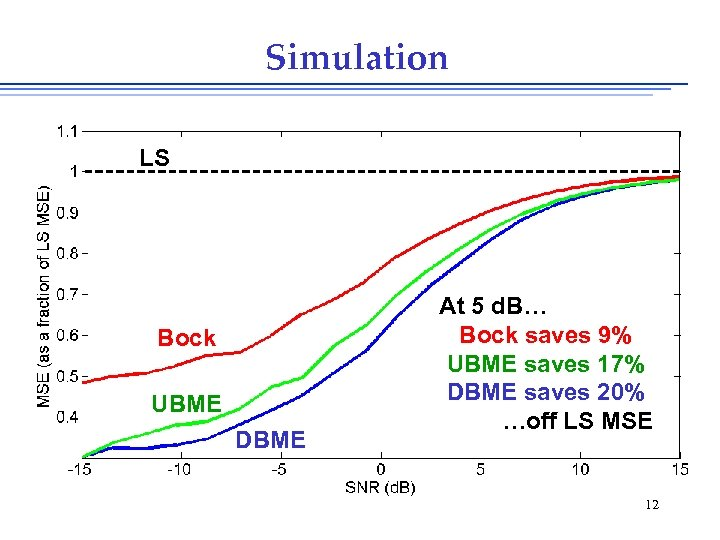Simulation LS Bock UBME DBME At 5 d. B… Bock saves 9% UBME saves 17% DBME saves 20% …off LS MSE 12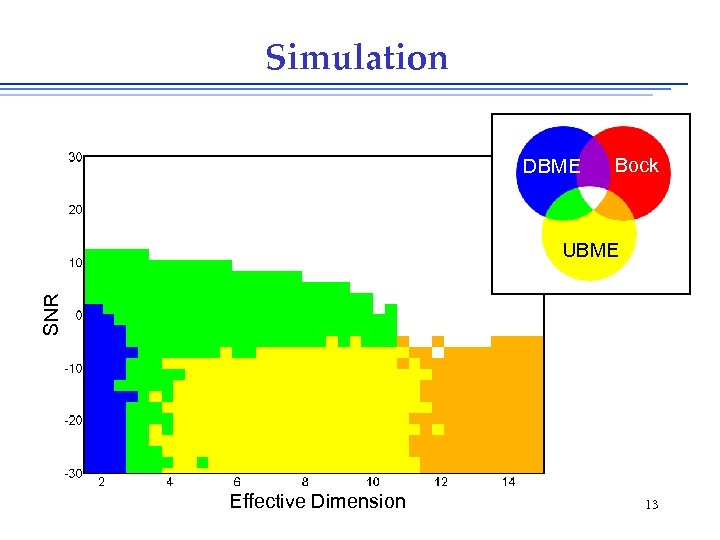Simulation DBME Bock SNR UBME Effective Dimension 13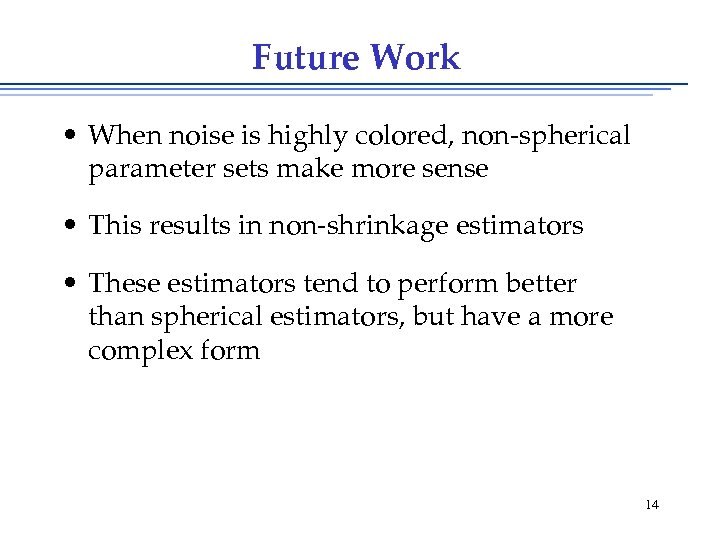Future Work • When noise is highly colored, non-spherical parameter sets make more sense • This results in non-shrinkage estimators • These estimators tend to perform better than spherical estimators, but have a more complex form 14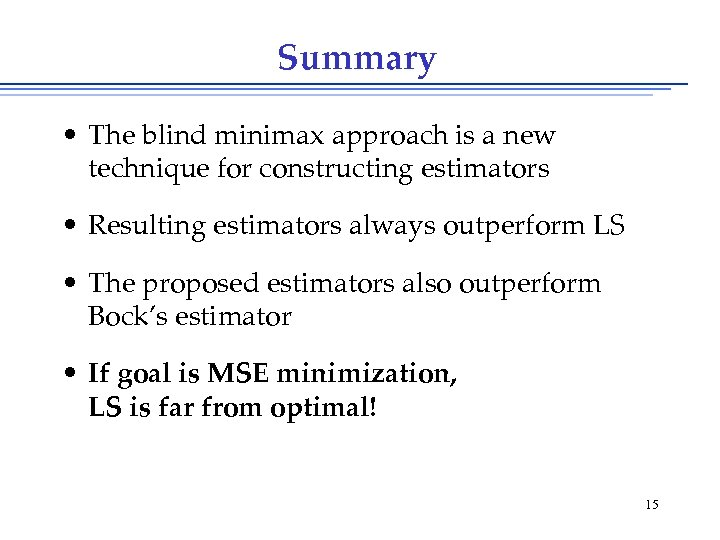Summary • The blind minimax approach is a new technique for constructing estimators • Resulting estimators always outperform LS • The proposed estimators also outperform Bock’s estimator • If goal is MSE minimization, LS is far from optimal! 15Thank you for your attention!